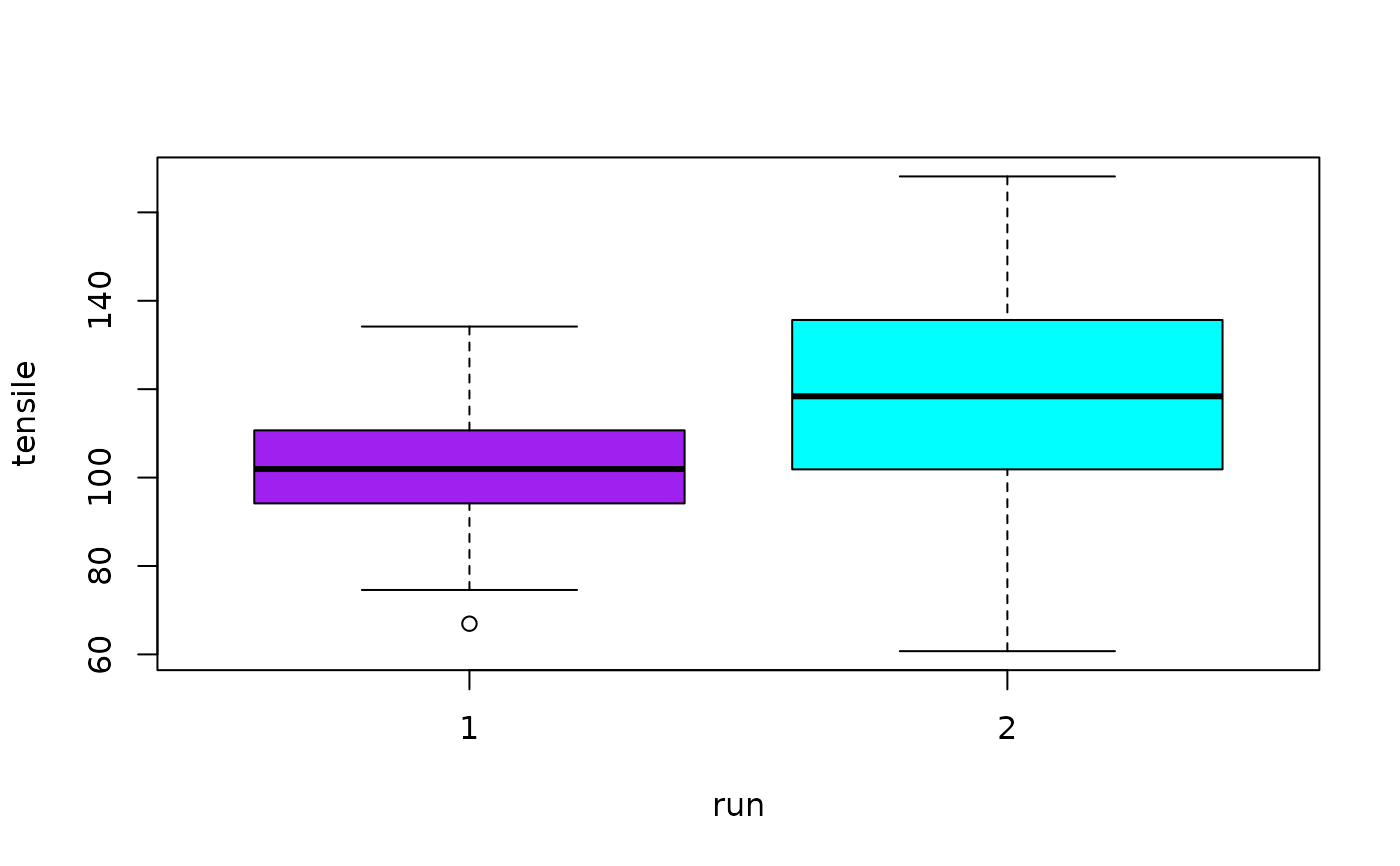Data for Example 7.11

Tensile

## Format

A data frame/tibble with 72 observations on two variables

tensile

plastic bag tensile strength (pounds per square inch)

run

factor with run number (1 or 2)

## Examples


boxplot(tensile ~ run, data = Tensile,
col = c("purple", "cyan"))t.test(tensile ~ run, data = Tensile)
#>
#> 	Welch Two Sample t-test
#>
#> data:  tensile by run
#> t = -3.4505, df = 64.136, p-value = 0.0009946
#> alternative hypothesis: true difference in means is not equal to 0
#> 95 percent confidence interval:
#>  -25.029593  -6.674665
#> sample estimates:
#> mean in group 1 mean in group 2
#>        102.3346        118.1868
#>